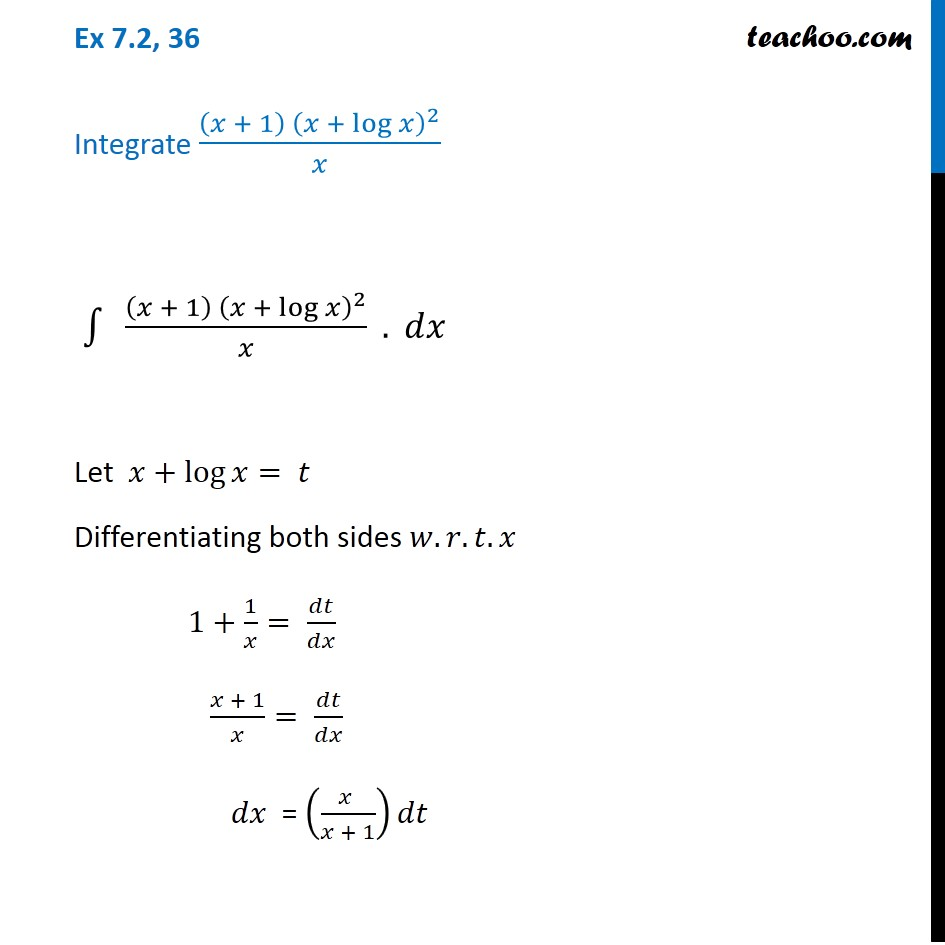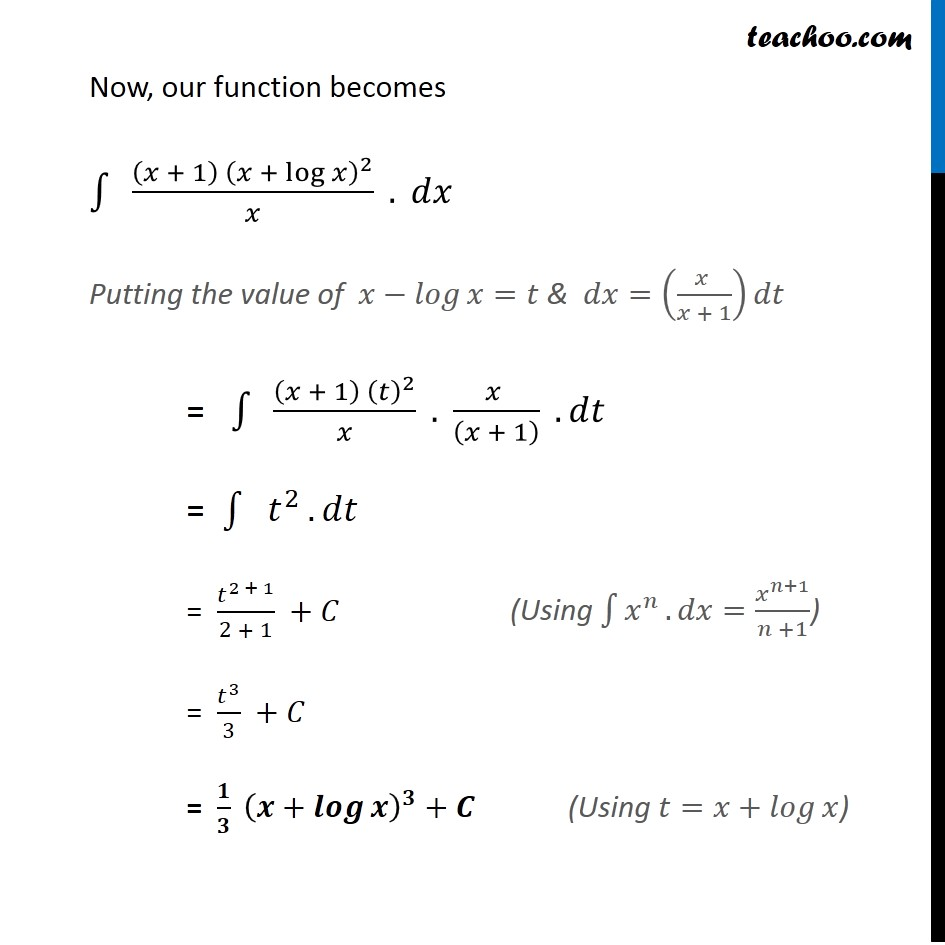1. Chapter 7 Class 12 Integrals (Term 2)
2. Concept wise
3. Integration by substitution - lnx

Transcript

Ex 7.2, 36 Integrate ((𝑥 + 1) (𝑥 + log⁡𝑥 )^2)/𝑥 ∫1▒〖" " ((𝑥 + 1) (𝑥 + log⁡𝑥 )^2)/𝑥〗 . 𝑑𝑥 Let 𝑥+log⁡𝑥= 𝑡 Differentiating both sides 𝑤.𝑟.𝑡.𝑥 1+1/𝑥= 𝑑𝑡/𝑑𝑥 (𝑥 + 1)/𝑥= 𝑑𝑡/𝑑𝑥 " " 𝑑𝑥 = ((𝑥 )/(𝑥 + 1))𝑑𝑡 Now, our function becomes ∫1▒〖" " ((𝑥 + 1) (𝑥 + log⁡𝑥 )^2)/𝑥〗 . 𝑑𝑥 Putting the value of 𝑥−𝑙𝑜𝑔⁡𝑥=𝑡 & 𝑑𝑥=((𝑥 )/(𝑥 + 1))𝑑𝑡 = ∫1▒〖" " ((𝑥 + 1) (𝑡)^2)/𝑥〗 . (𝑥 )/((𝑥 + 1) ) . 𝑑𝑡 = ∫1▒〖" " 𝑡^2 〗. 𝑑𝑡" " = 𝑡^(2 + 1)/(2 + 1) +𝐶 = 𝑡^3/3 +𝐶 = 𝟏/𝟑 (𝒙+𝒍𝒐𝒈⁡𝒙 )^𝟑+𝑪 (Using ∫1▒𝑥^𝑛 . 𝑑𝑥=𝑥^(𝑛+1)/(𝑛 +1)) (Using 𝑡=𝑥+𝑙𝑜𝑔⁡𝑥)

Integration by substitution - lnx

Chapter 7 Class 12 Integrals (Term 2)
Concept wise